Documentation

# impulseest

Nonparametric impulse response estimation

## Syntax

```sys = impulseest(data) sys = impulseest(data,N) sys = impulseest(data,N,NK) sys = impulseest(___,options) ```

## Description

`sys = impulseest(data)` estimates an impulse response model, `sys`, using time- or frequency-domain data, `data`. The model order (number of nonzero impulse response coefficients) is determined automatically using persistence of excitation analysis on the input data.

`sys = impulseest(data,N)` estimates an `N`th order impulse response model, corresponding to the time range 0 :Ts : (N –1)*Ts, where `Ts` is the data sample time.

`sys = impulseest(data,N,NK)` specifies a transport delay of `NK` samples in the estimated impulse response.

`sys = impulseest(___,options)` specifies estimation options using the options set `options`.

Use nonparametric impulse response to analyze `data` for feedback effects, delays and significant time constants.

## Input Arguments

 `data` Estimation data with at least one input signal and nonzero sample time. For time domain estimation, `data` is an `iddata` object containing the input and output signal values. For frequency domain estimation, `data` can be one of the following: Frequency response data (`frd` or `idfrd`)`iddata` object with its properties specified as follows:`InputData` — Fourier transform of the input signal`OutputData` — Fourier transform of the output signal`Domain` — ‘Frequency’ `N` Order of the FIR model. Must be one of the following: A positive integer.For data containing Nu inputs and Ny outputs, you can also specify N as an Ny-by-Nu matrix of positive integers, such that N(i,j) represents the length of impulse response from input j to output i.`[]` — Determines the order automatically using persistence of excitation analysis on the input data. `NK` Transport delay in the estimated impulse response, specified as a scalar integer. For data containing Nu inputs and Ny outputs, you can also specify a Ny-by-Nu matrix. To generate the impulse response coefficients for negative time values, which is useful for feedback analysis, use a negative integer. If you specify a negative value, the value must be the same across all output channels.You can also use `NK = 'negative'` to automatically pick negative lags for all input/output channels of the model.Specify `NK = 0` if the delay is unknown. The true delay is then be indicated by insignificant impulse response values in the beginning of the response.Specify `NK = 1` to create a system whose leading numerator coefficient is zero. Positive values of `NK` greater than 1 are stored in the `IODelay` property of `sys` (```sys.IODelay = max(NK-1,0)```). Negative values are stored in the `InputDelay` property. The impulse response (input `j` to output `i`) coefficients correspond to the time span ```NK(i,j)*Ts : Ts : (N(ij)+NK(i,j)-1)*Ts```. Default: zeros(Ny, Nu) `options` Estimation options that specify the following: Prefilter orderRegularization algorithmInput and output data offsets Use `impulseestOptions` to create the options set.

## Output Arguments

`sys`

Estimated impulse response model, returned as an `idtf` model, which encapsulates an FIR model.

Information about the estimation results and options used is stored in the `Report` property of the model. ` Report` has the following fields:

Report FieldDescription
`Status`

Summary of the model status, which indicates whether the model was created by construction or obtained by estimation.

`Method`

Estimation command used.

`Fit`

Quantitative assessment of the estimation, returned as a structure. See Loss Function and Model Quality Metrics for more information on these quality metrics. The structure has the following fields:

FieldDescription
`FitPercent`

Normalized root mean squared error (NRMSE) measure of how well the response of the model fits the estimation data, expressed as a percentage.

`LossFcn`

Value of the loss function when the estimation completes.

`MSE`

Mean squared error (MSE) measure of how well the response of the model fits the estimation data.

`FPE`

Final prediction error for the model.

`AIC`

Raw Akaike Information Criteria (AIC) measure of model quality.

`AICc`

Small sample-size corrected AIC.

`nAIC`

Normalized AIC.

`BIC`

Bayesian Information Criteria (BIC).

`Parameters`

Estimated values of model parameters.

`OptionsUsed`

Option set used for estimation. If no custom options were configured, this is a set of default options. See `impulseestOptions` for more information.

`RandState`

State of the random number stream at the start of estimation. Empty, `[]`, if randomization was not used during estimation. For more information, see `rng` in the MATLAB® documentation.

`DataUsed`

Attributes of the data used for estimation, returned as a structure with the following fields:

FieldDescription
`Name`

Name of the data set.

`Type`

Data type.

`Length`

Number of data samples.

`Ts`

Sample time.

`InterSample`

Input intersample behavior, returned as one of the following values:

• `'zoh'` — Zero-order hold maintains a piecewise-constant input signal between samples.

• `'foh'` — First-order hold maintains a piecewise-linear input signal between samples.

• `'bl'` — Band-limited behavior specifies that the continuous-time input signal has zero power above the Nyquist frequency.

`InputOffset`

Offset removed from time-domain input data during estimation. For nonlinear models, it is `[]`.

`OutputOffset`

Offset removed from time-domain output data during estimation. For nonlinear models, it is `[]`.

For more information on using `Report`, see Estimation Report.

## Examples

collapse all

Compute a nonparametric impulse response model using data from a hair dryer. The input is the voltage applied to the heater and the output is the heater temperature. Use the first 500 samples for estimation.

```load dry2 ze = dry2(1:500); sys = impulseest(ze);```

`ze` is an `iddata` object that contains time-domain data. `sys`, the identified nonparametric impulse response model, is an `idtf` model.

Analyze the impulse response of the identified model from time 0 to 1.

`h = impulseplot(sys,1);`Right-click the plot and select Characteristics > Confidence Region to view the statistically zero-response region. Alternatively, you can use the `showConfidence` command.

`showConfidence(h);`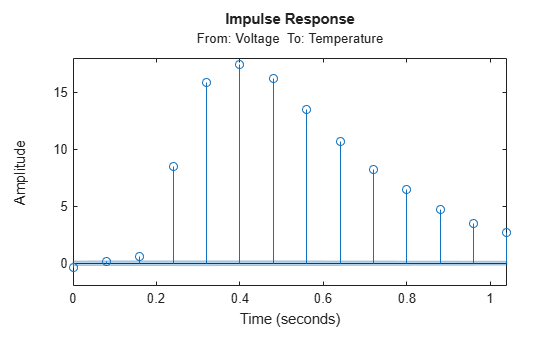The first significantly nonzero response value occurs at 0.24 seconds, or, the third lag. This implies that the transport delay is 3 samples. To generate a model where the 3-sample delay is imposed, set the transport delay to 3:

`sys = impulseest(ze,[],3) `

`load iddata3 z3;`

Estimate a 35th order FIR model.

`sys = impulseest(z3,35);`

Estimate an impulse response model with transport delay of 3 samples.

If you know about the presence of delay in the input/output data in advance, use the value as a transport delay for impulse response estimation.

Generate data with 3-sample input to output lag. Create a random input signal and use an `idpoly` model to simulate the output data.

```u = rand(100,1); sys = idpoly([1 .1 .4],[0 0 0 4 -2],[1 1 .1]); opt = simOptions('AddNoise',true); y = sim(sys,u,opt); data = iddata(y,u,1);```

Estimate a 20th order model with a 3-sample transport delay.

`model = impulseest(data,20,3);`

Obtain regularized estimates of impulse response model using the regularizing kernel estimation option.

Estimate a model using regularization.

```load iddata3 z3; sys1 = impulseest(z3);```

By default, tuned and correlated kernel (`'TC'`) is used for regularization.

Estimate a model with no regularization.

```opt = impulseestOptions('RegularizationKernel','none'); sys2 = impulseest(z3,opt);```

Compare the impulse response of both models.

`h = impulseplot(sys1,sys2,70);`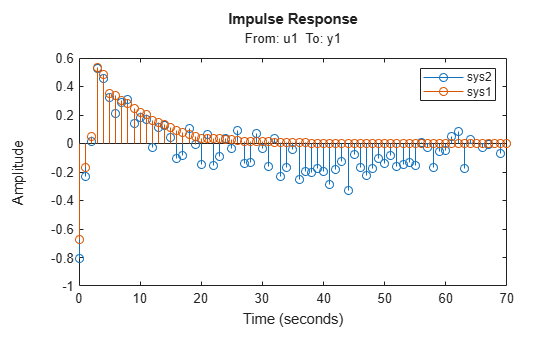As the plot shows, using regularization makes the response smoother.

Plot the confidence interval.

`showConfidence(h);`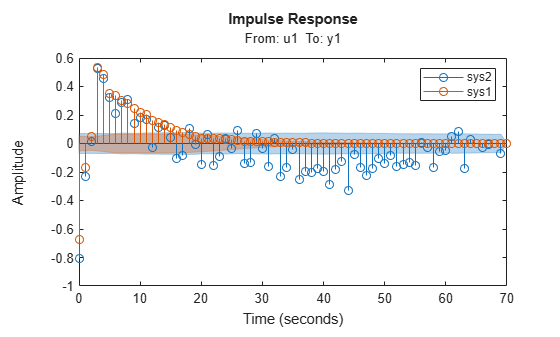The uncertainty in the computed response is reduced at larger lags for the model using regularization. Regularization decreases variance at the price of some bias. The tuning of the regularization is such that the bias is dominated by the variance error though.

`load regularizationExampleData eData;`

Create a transfer function model used for generating the estimation data (true system).

`trueSys = idtf([0.02008 0.04017 0.02008],[1 -1.561 0.6414],1);`

Obtain regularized impulse response (FIR) model.

```opt = impulseestOptions('RegularizationKernel','DC'); m0 = impulseest(eData,70,opt);```

Convert the model into a state-space model and reduce the model order.

`m1 = balred(idss(m0),15);`

Obtain a second state-space model using regularized reduction of an ARX model.

`m2 = ssregest(eData,15);`

Compare the impulse responses of the true system and the estimated models.

```impulse(trueSys,m1,m2,50); legend('trueSys','m1','m2');```Use the empirical impulse response of the measured data to verify whether there are feedback effects. Significant amplitude of the impulse response for negative time values indicates feedback effects in data.

Compute the noncausal impulse response using a fourth-order prewhitening filter, automatically chosen order and negative lag using nonregularized estimation.

```load iddata3 z3; opt = impulseestOptions('pw',4,'RegularizationKernel','none'); sys = impulseest(z3,[],'negative',opt);```

`sys` is a noncausal model containing response values for negative time.

Analyze the impulse response of the identified model.

`h = impulseplot(sys);`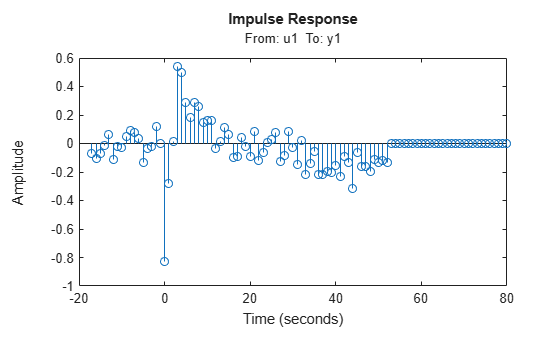View the statistically zero-response region by right-clicking on the plot and selecting Characteristics > Confidence Region. Alternatively, you can use the `showConfidence` command.

`showConfidence(h);`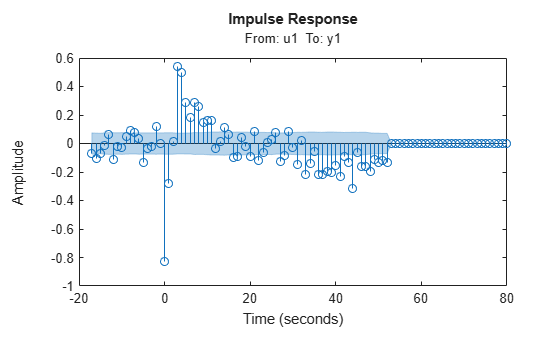The large response value at `t=0` (zero lag) suggests that the data comes from a process containing feedthrough. That is, the input affects the output instantaneously. There could also be a direct feedback effect (proportional control without some delay that `u(t)` is determined partly by `y(t)`).

Also, the response values are significant for some negative time lags, such as at -7 seconds and -9 seconds. Such significant negative values suggest the possibility of feedback in the data.

Compute an impulse response model for frequency response data.

```load demofr; zfr = AMP.*exp(1i*PHA*pi/180); Ts = 0.1; data = idfrd(zfr,W,Ts); sys = impulseest(data);```

Identify parametric and nonparametric models for a data set, and compare their step response.

Identify the impulse response model (nonparametric) and state-space model (parametric), based on a data set.

```load iddata1 z1; sys1 = impulseest(z1); sys2 = ssest(z1,4);```

`sys1` is a discrete-time identified transfer function model. `sys2` is a continuous-time identified state-space model.

Compare the step response for `sys1` and `sys2`.

```step(sys1,'b',sys2,'r'); legend('impulse response model','state-space model');```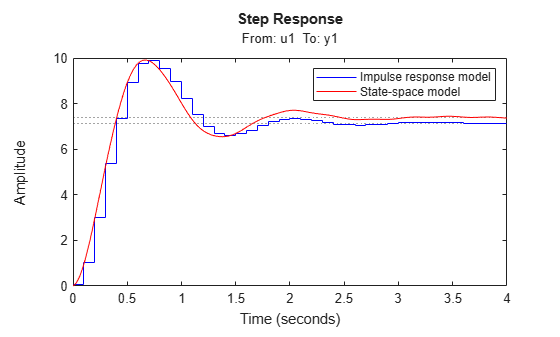## Tips

• To view the impulse or step response of `sys`, use either `impulseplot` or `stepplot`, respectively.

• A significant value of the impulse response of `sys` for negative time values indicates the presence of feedback in the data.

• To view the region of insignificant impulse response (statistically zero) in a plot, right-click on the plot and select Characteristics > Confidence Region. A patch depicting the zero-response region appears on the plot. The impulse response at any time value is significant only if it lies outside the zero response region. The level of significance depends on the number of standard deviations specified in `showConfidence` or options in the property editor. A common choice is 3 standard deviations, which gives 99.7% significance.

## Algorithms

Correlation analysis refers to methods that estimate the impulse response of a linear model, without specific assumptions about model orders.

The impulse response, g, is the system's output when the input is an impulse signal. The output response to a general input, u(t), is obtained as the convolution with the impulse response. In continuous time:

`$y\left(t\right)={\int }_{-\infty }^{t}g\left(\tau \right)u\left(t-\tau \right)d\tau$`

In discrete-time:

`$y\left(t\right)=\sum _{k=1}^{\infty }g\left(k\right)u\left(t-k\right)$`

The values of g(k) are the discrete time impulse response coefficients.

You can estimate the values from observed input-output data in several different ways. `impulseest` estimates the first n coefficients using the least-squares method to obtain a finite impulse response (FIR) model of order n.

Several important options are associated with the estimate:

• Prewhitening — The input can be pre-whitened by applying an input-whitening filter of order `PW` to the data. This minimizes the effect of the neglected tail (`k > n`) of the impulse response.

1. A filter of order `PW` is applied such that it whitens the input signal `u`:

`1/A = A(u)e`, where `A` is a polynomial and `e` is white noise.

2. The inputs and outputs are filtered using the filter:

`uf = Au`, `yf = Ay`

3. The filtered signals `uf` and `yf` are used for estimation.

You can specify prewhitening using the `PW` name-value pair argument of `impulseestOptions`.

• Regularization — The least-squares estimate can be regularized. This means that a prior estimate of the decay and mutual correlation among `g(k)` is formed and used to merge with the information about `g` from the observed data. This gives an estimate with less variance, at the price of some bias. You can choose one of the several kernels to encode the prior estimate.

This option is essential because, often, the model order `n` can be quite large. In cases where there is no regularization, `n` can be automatically decreased to secure a reasonable variance.

You can specify the regularizing kernel using the `RegularizationKernel` Name-Value pair argument of `impulseestOptions`.

• Autoregressive Parameters — The basic underlying FIR model can be complemented by `NA` autoregressive parameters, making it an ARX model.

`$y\left(t\right)=\sum _{k=1}^{n}g\left(k\right)u\left(t-k\right)-\sum _{k=1}^{NA}{a}_{k}y\left(t-k\right)$`

This gives both better results for small `n` and allows unbiased estimates when data are generated in closed loop. `impulseest` uses NA = 5 for t>0 and NA = 0 (no autoregressive component) for t<0.

• Noncausal effects — Response for negative lags. It may happen that the data has been generated partly by output feedback:

`$u\left(t\right)=\sum _{k=0}^{\infty }h\left(k\right)y\left(t-k\right)+r\left(t\right)$`

where h(k) is the impulse response of the regulator and r is a setpoint or disturbance term. The existence and character of such feedback h can be estimated in the same way as g, simply by trading places between y and u in the estimation call. Using `impulseest` with an indication of negative delays, , returns a model `mi` with an impulse response

`$\left[h\left(-nk\right),h\left(-nk-1\right),...,h\left(0\right),g\left(1\right),g\left(2\right),...,g\left(nb+nk\right)\right]$`

aligned so that it corresponds to lags $\left[nk,nk+1,..,0,1,2,...,nb+nk\right]$. This is achieved because the input delay (`InputDelay`) of model `mi` is `nk`.

For a multi-input multi-output system, the impulse response g(k) is an ny-by-nu matrix, where ny is the number of outputs and nu is the number of inputs. The ij element of the matrix g(k) describes the behavior of the ith output after an impulse in the jth input.